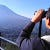Codility — CyclicRotation — Swift

public func solution(_ A : inout [Int], _ K : Int) -> [Int] {
if A.count == K || K == 0 || A.count == 0 { return A }
let rotation = K % A.count
let range = 0...(A.count - 1 - rotation)
let firstPick = A[range]
A.removeSubrange(range) …

RxSwift Handle Error Gracefully?

button.rx.tap
.flatMapLatest {
API.index()
}
.subscribe()
.disposed(by: bag)
.flatMapLatest {
API.index()
.catchError { _ in Observable.empty() }
}